## From the hen to the egg

### Case and control studies

Surely someone overflowing genius has asked you on any occasion, with a smug look, what came first, the hen or the egg? Well, the next time you meet with someone like this, you can answer with another question: what is it that that the hen and the egg have something to do which each other? Because we must first now not only whether if to have hens we have to have eggs before, but also how likely is to end having hens, with or without eggs (some twisted mind will say that the question could be raised backwards, but I am among those to think that the first thing we have to have, no offense, are eggs).

#### What is a case and control study?

This approach would lead us to the design of a case-control study, which is an observational and analytical study in which sampling is done on the basis of presenting a certain disease or effect (the cases) and that group is compared with another group that it does not present it (the controls), in order to determine if there is a difference in the frequency of exposure to a certain risk factor between the two groups. These studies are of retrograde directionality and of mixed temporality, so most of them are retrospective, although, as was the case with cohort studies, they can also be prospective (perhaps the most useful key to distinguish between the two is the sampling of each one, based on the exposure in the cohort studies and based on the effect in the cases and controls).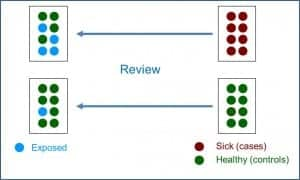In the attached figure you can see the typical design of a case-control study. These studies are based on a specific population from which a sample of cases that usually includes all diagnosed and available cases, are compared with a control group consisting of a balanced sample of healthy subjects from the same population. However, it is increasingly common to find variations in the basic design that combine characteristics of the cohort and case-control studies, comparing the cases that appear in a stable cohort over time with controls of a partial sample extracted from that same cohort.

#### Design’s variations

The best known of this type of mixed designs is that of nested in a cohort cases and controls. In these cases, we start with a well-known cohort in which we identify the cases that are occurring. Each time a case appears, it is paired with one or more controls also taken from the initial cohort. If we think about it briefly, it is possible that a subject that is initially selected as a control becomes a case over time (develop the disease under study). Although it may seem that this may bias the results, this should not be the case, since it is about measuring the effect of the exposure at the time of the analysis. This design can be done with smaller cohorts, so it can be simpler and cheaper. In addition, it is especially useful in very dynamic cohorts with many inputs and outputs over time, especially if the incidence of the disease under study is low.

Another variant of the basic design are the cohort and cases studies. In this type, we initially have a very large cohort from which we will select a smaller sub-cohort. The cases will be the patients that are produced in either of the two cohorts, while the controls will be the subjects of the smallest (and most manageable) sub-cohort. These studies have a method of analysis a little more complicated than the basic designs, since they have to compensate the fact that the cases are overrepresented because they come from the two cohorts. The great advantage of this design is that it allows studying several diseases at the same time, comparing the different cohorts of patients with the sub-cohort chosen as control.

Finally, one last variation that we are going to discuss is that of the polysemic case-cohort studies, also known as crossed cases and controls, also known as self-controlled cases. In this paired design, each individual serves as their own control, comparing the exposure during the period of time closest to the onset of the disease (case period) with the exposure during the previous period of time (control period). This study approach is useful when the exposure is short, with a foreseeable time of action and produces a disease of short duration in time. They are widely used, for example, to study the adverse effects of vaccines.

#### Association measure: odds ratio

As in cohort studies, case-control studies allow the calculation of a whole series of association and impact measures. Of course, here we have a fundamental difference with cohort studies. In cohort studies we started from a cohort without patients in which the patients appeared during the follow-up, which allowed us to calculate the risk of becoming ill over time (incidence). Thus, the quotient between incidents of exposed and not exposed gave us the risk ratio, the main measure of association.

However, as can be deduced from the design of case-control studies, in these cases we cannot make a direct estimate of the incidence or prevalence of the disease, since the proportion of patients is determined by the selection criteria of the researcher and not by the incidence in the population (a fixed number of cases and controls are selected at the beginning, but we cannot calculate the risk of being a case in the population). Thus, before the impossibility of calculating the risk ratio, we will resort to the calculation of the odds ratio (OR), as you can see in the second figure.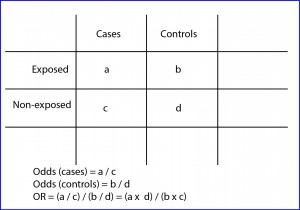The OR has a similar interpretation that the risk ratio, being able to value from zero to infinity. An OR = 1 means that there is no association between exposure and effect. An OR <1 means that exposure is a factor of protection against the effect. Finally, an OR> 1 indicates that the exposure is a risk factor, the higher the value of the OR.

Anyway and only for those who like getting into trouble, I will tell you that it is possible to calculate the incidence rates from the results of a case-control study. If the incidence of the disease under study is low (below 10%), OR and risk ratio can be comparable, so we can estimate the incidence in an approximate way. If the incidence of the disease is greater, the OR tends to overestimate the risk ratio, so we cannot consider them to be equivalent. In any case, in these cases, if we previously know the incidence of the disease in the population (obtained from other studies), we can calculate the incidence using the following formulas:

I0 = It / (OR x Pe) + P0

Ie = I0 x OR,

where It is the total incidence, Ie the incidence in exposed, I0 the incidence in not exposed, Pe the proportion of exposed, and P0 the proportion of not exposed.

Although the OR allows estimating the strength of the association between the exposure and the effect, it does not report on the potential effect that eliminating the exposure on the health of the population would have. For this, we will have to resort to the measures of attributable risk (as we did with cohort studies), which can be absolute or relative.

#### Impact measures

There are two absolute measures of attributable risk. The first is the attributable risk in exposed (ARE), which is the difference between the incidence in exposed and not exposed and represents the amount of incidence that can be attributed to the risk factor in the exposed. The second is the population attributable risk (PAR), which represents the amount of incidence that can be attributed to the risk factor in the general population.

On the other hand, there are also two relative measures of attributable risk (also known as proportions or attributable or etiological fractions). First, the attributable fraction in exposed (AFE), which represents the difference of risk relative to the incidence in the group of exposed to the factor. Second, the population attributable fraction (PAF), which represents the difference in risk relative to the incidence in the general population.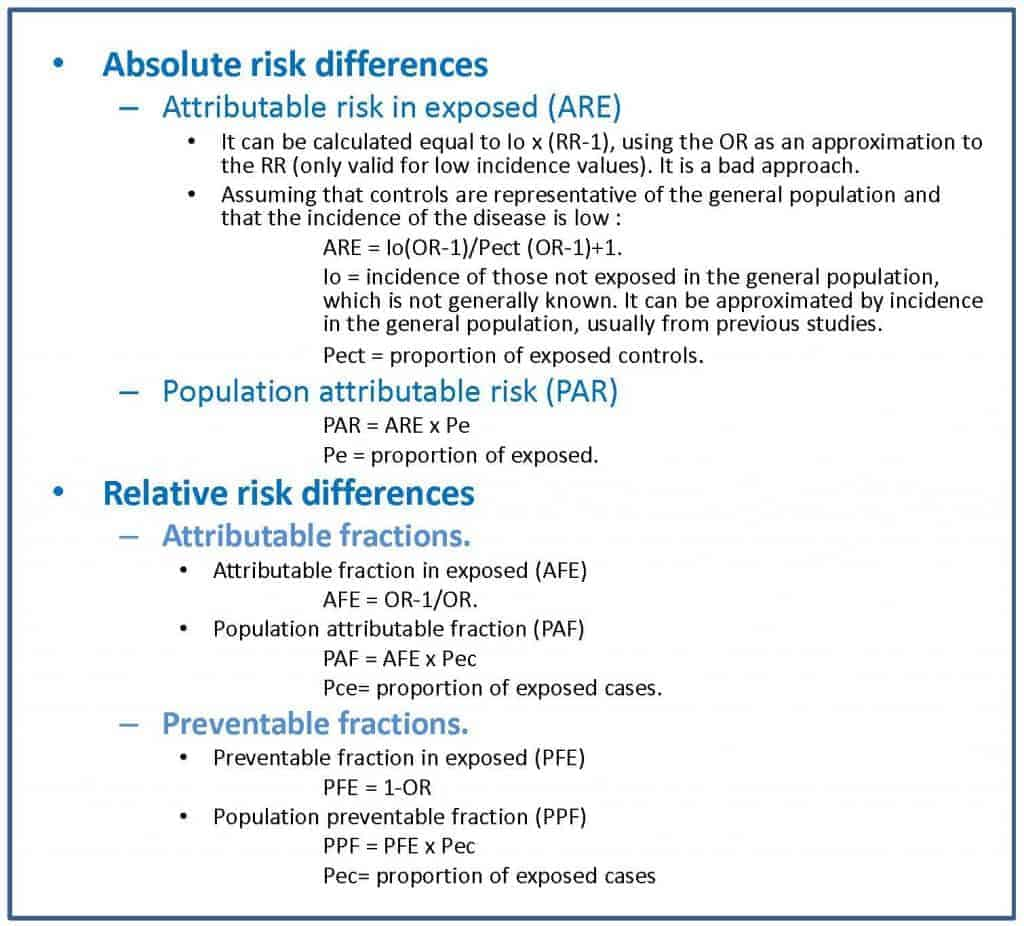In the attached table I show you the formulas for the calculation of these parameters, which is somewhat more complex than in the case of cohort studies.

The problem with these impact measures is that they can sometimes be difficult for the clinician to interpret. For this reason, and inspired by the calculation of the number needed to treat (NNT) of clinical trials, a series of measures called impact numbers have been devised, which give us a more direct idea of the effect of the exposure factor on the disease. in study. These impact numbers are the number of impact in exposed (NIE), the number of impact in cases (NIC) and the number of impact in exposed cases (NIEC).

Let’s start with the simplest one. The NIE would be the equivalent of the NNT and would be calculated as the inverse of the absolute risk reduction or of the risk difference between exposed and not exposed. The NNT is the number of people who should be treated to prevent a case compared to the control group. The NIE represents the average number of people who have to be exposed to the risk factor so that a new case of illness occurs compared to the people who are not exposed. For example, a NIE of 10 means that out of every 10 exposed there will be a case of disease attributable to the risk factor studied.

The NIC is the inverse of the PAF, so it defines the average number of sick people among which a case is due to the risk factor. An NIC of 10 means that for every 10 patients in the population, one is attributable to the risk factor under study.

Finally, the NIEC is the inverse of the AFE. It is the average number of patients among which a case is attributable to the risk factor.

In summary, these three parameters measure the impact of exposure among all exposed (NIE), among all patients (NIC) and among all patients who have been exposed (NIEC). It will be useful for us to try to calculate them if the authors of the study do not do so, since they will give us an idea of the real impact of the exposure on the effect. In the second table I show you the formulas that you can use to obtain them.

As a culmination to the previous three, we could estimate the effect of the exposure on the entire population by calculating the number of impact on the population (NIP), for which we have only to do the inverse of the ARP. Thus, a NIP of 3000 means that for every 3,000 subjects of the population there will be a case of illness due to exposure.

#### Bias of case and control studies

In addition to assessing the measures of association and impact, when appraising a case-control study we will have to pay special attention to the presence of biases, since they are the observational studies that have the greatest risk of presenting them.

Case-control studies are relatively simple to make, have in general lower cost than other observational studies (including cohort studies), allow us to study various exposure factors at the same time and to know how they interact, and they are ideal for diseases of exposure factors with very low frequency. The problem with this type of design is that you have to be extremely careful selecting cases and controls, as it is very easy to commit a list of biases that, to this day, does not have a known end.

In general, the selection criteria should be the same for cases and controls, but as to be a case one has to be diagnosed and be available for the study, it’s very likely that cases are not fully representative of the population. For example, if the diagnostic criteria are not sensitive and specific enough we’ll get many false positives and negatives, with the consequent dilution of the effect of the exposure to the factor.

Other possible problem depends on the selection of incident (newly diagnosed) or prevalent cases. Prevalence based studies favor the selection of the survivors (as far as it’s known, no dead has agreed to participate in any study) and if survival is related to the exposure, the risk identified will be less than with incident cases. This effect is even more evident when the exposure factor is of good prognosis, a situation in which prevalence studies produces a greater overestimation of the association. As an example to better understand these issues, let’s suppose that the risk of suffer a stroke is higher the more one smokes. If we include only prevalent cases we’ll exclude the people dead of more severe heart attacks, which probably would be the one who smoke most, with which the effect of smoking could be underestimated.

But if selecting cases seems complicated, it’s nothing compared to a good selection of controls. Ideally, controls have had the same likely of exposure than cases or, put it another way, should be representative of the population from which the cases were extracted. In addition, this must be combined with the exclusion of those who have any illness related positively or negatively to the exposure factor. For example, If we want to waste our time and study the association between air passengers who have thrombophlebitis and prior aspirin ingestion, we must exclude from the study the controls that have any other disease being treated with aspirin, even if they had not taken it before the journey.

We have also to be careful with some habits of control selection. For instance, patients who go to the hospital for reasons different to that of study are at hand and tend to be very cooperative and, being sick, they surely better recall past exposure to risk factors. But the problem is that they are ill, so the pattern of exposure to risk factors can be different to the general population.

Another resource is to include neighbors, friend, relatives, etc. These usually are very comparable and cooperative, but we have the risk that there’re paired exposure habit that can alter study results. These entire problems are avoided taking controls from general population, but it is more costly in effort and money, they usually are less cooperative and, above all, much more forgetful (healthy people recall less about past exposures to risk factors), with so the quality of the information we obtain from cases and controls can be very different.

#### We’re leaving…

Just one more comment to end this theme so enjoyable. Case-control studies share a characteristic with the rest of the observational studies: they detect the association between exposure and effect, but they do not allow us to establish causality relations with certainty, for which we need other types of studies such as randomized clinical trials. But that is another story…

### Cohort studies

What a fellows, those Romans!. They came, they saw and they conquered. With those legions, each one with ten cohorts, each cohort with almost five hundred Romans with their skirts and strappy sandals. The cohorts were groups of soldier that were in reach of the speech of the same boss. They always went forward, never retreating. This is how you can conquer Gaul (though not entirely, as is well known).

#### Cohort studies characteristics

In epidemiology, a cohort is also a group of people who share something, but instead of being the boss’s harangue it is the exposure to a factor that is studied over time (neither the skirt nor the sandals are essential) . Thus, a cohort study is a type of observational, analytical design, of anterograde directionality and of concurrent or mixed temporality that compares the frequency with which a certain effect occurs (usually a disease) in two different groups (cohorts), one of them exposed to one factor and another not exposed to the same factor (see attached figure).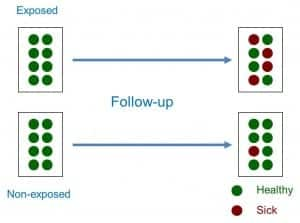Therefore, sampling is related to exposure to the factor. Both cohorts are studied over time, which is why most of the cohort studies are prospective or of concurrent temporality (they go forward, like the Roman cohorts). However, it is possible to do retrospective cohort studies once both the exposure and the effect have occurred. In these cases, the researcher identifies the exposure in the past, reconstructs the experience of the cohort over time and attends in the present to the appearance of the effect, which is why they are studies of mixed temporality.

We can also classify the cohort studies according to whether they use an internal or external comparison group. Sometimes we can use two internal cohorts belonging to the same general population, classifying the subjects in one or another cohort according to the level of exposure to the factor. However, other times the exposed cohort will interest us because of its high level of exposure, so we will prefer to select an external cohort of subjects not exposed to make the comparison between both.

Another important aspect when classifying the cohort studies is the time of inclusion of the subjects in the study. When we only select the subjects that meet the inclusion criteria at the beginning of the study, we speak of a fixed cohort, whereas we will speak of an open or dynamic cohort when subjects continue to enter the study throughout the follow-up. This aspect will be important, as we will see later, when calculating the association measures between exposure and effect.

Finally, and as a curiosity, we can also do a study with a single cohort if we want to study the incidence or evolution of a certain disease. Although we can always compare the results with other known data of the general population, this type of designs lacks a comparison group in the strict sense, so it is included within the longitudinal descriptive studies.

When followed up over time, the cohort studies allow us to calculate the incidence of the effect between exposed and not exposed, calculating from them a series of association measures and specific impact measures.

#### Association measures in cohort studies

In studies with closed cohorts in which the number of participants does not change, the measure of association is the (RR), which is the ratio between the incidence of exposed (Ie) and unexposed (I0): RR = Ie / I0.

As we know, the RR can value from 0 to infinity. A RR = 1 means that there is no association between exposure and effect. A RR <1 means that exposure is a factor of protection against the effect. Finally, a RR> 1 indicates that exposure is a risk factor, the greater the value of the RR.

The case of studies with open cohorts in which participants can enter and leave during the follow-up is a bit more complex, since instead of incidences we will calculate incidence densities, a term that refers to the number of cases of the effect or disease that they occur referring to the number of people followed by each follow-up time (for example, number of cases per 100 person-years). In these cases, instead of the RR we will calculate the incidence density ratio, which is the quotient of the incidence density in exposed divided by the density in not exposed.

#### Impact measures in cohort studies

These measures allow us to estimate the strength of the association between the exposure to the factor and the effect, but they do not inform us about the potential impact that exposure has on the health of the population (the effect that eliminating this factor would have on the health of the population). For this, we will have to resort to the measures of attributable risk, which can be absolute or relative.

There are two absolute measures of attributable risk. The first is the attributable risk in exposed (ARE), which is the difference between the incidence in exposed and not exposed and represents the amount of incidence that can be attributed to the risk factor in the exposed. The second is the population attributable risk (PAR), which represents the amount of incidence that can be attributed to the risk factor in the general population.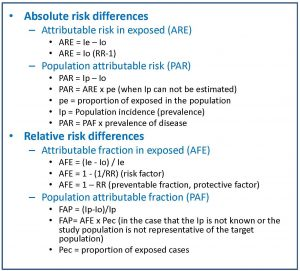On the other hand, there are also two relative measures of attributable risk (also known as proportions or attributable or etiological fractions). First, the attributable fraction in exposed (AFE), which represents the difference of risk relative to the incidence in the group of exposed to the factor. Second, the population attributable fraction (PAF), which represents the difference in risk relative to the incidence in the general population.

In the attached table you can see the formulas that are used for the calculation of these impact measures.

The problem with these impact measures is that they can sometimes be difficult for the clinician to interpret. For this reason, and inspired by the calculation of the number needed to treat (NNT) of clinical trials, a series of measures called impact numbers have been devised, which give us a more direct idea of the effect of the exposure factor on the disease. in study. These impact numbers are the number of impact in exposed (NIE), the number of impact in cases (NIC) and the number of impact in exposed cases (NIEC).

Let’s start with the simplest one. The NIE would be the equivalent of the NNT and would be calculated as the inverse of the absolute risk reduction or of the risk difference between exposed and not exposed. The NNT is the number of people who should be treated to prevent a case compared to the control group. The NIE represents the average number of people who have to be exposed to the risk factor so that a new case of illness occurs compared to the people who are not exposed. For example, a NIE of 10 means that out of every 10 exposed there will be a case of disease attributable to the risk factor studied.

The NIC is the inverse of the PAF, so it defines the average number of sick people among which a case is due to the risk factor. An NIC of 10 means that for every 10 patients in the population, one is attributable to the risk factor under study.

Finally, the NIEC is the inverse of the AFE. It is the average number of patients among which a case is attributable to the risk factor.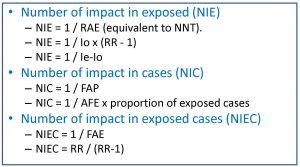In summary, these three parameters measure the impact of exposure among all exposed (NIE), among all patients (NIC) and among all patients who have been exposed (NIEC). It will be useful for us to try to calculate them if the authors of the study do not do so, since they will give us an idea of the real impact of the exposure on the effect. In the second table I show you the formulas that you can use to obtain them.

As a culmination to the previous three, we could estimate the effect of the exposure on the entire population by calculating the number of impact on the population (NIP), for which we have only to do the inverse of the ARP. Thus, a NIP of 3000 means that for every 3,000 subjects of the population there will be a case of illness due to exposure.

#### Bias in cohort studies

Another aspect that we must take into account when dealing with cohort studies is their risk of bias. In general, observational studies have a higher risk of bias than experimental studies, as well as being susceptible to the influence of confounding factors and effect modifying variables.

The selection bias must always be considered, since it can compromise the internal and external validity of the study results. The two cohorts should be comparable in all aspects, in addition to being representative of the population from which they come.

Another very typical bias of cohort studies is the classification bias, which occurs when an erroneous classification of the participants is made in terms of their exposure or the detection of the effect (basically, it is just another information bias). . The classification bias can be non-differential when the error occurs randomly independently of the study variables. This type of classification bias is in favor of the null hypothesis, that is, it makes it difficult for us to detect the association between exposure and effect, if it exists. If, despite the bias, we detect the association, then nothing bad will happen, but if we do not detect it, we will not know if it does not exist or if we do not see it because of the bad classification of the participants. On the other hand, the classification bias is differential when performed differently between the two cohorts and has to do with some of the study variables. In this case there is no forgiveness or possibility of amendment: the direction of this bias is unpredictable and mortally compromises the validity of the results.

Finally, we should always be alert to the possibility of confusion bias (due to confounding variables) or interaction bias (due to effect modifying variables). The ideal is to prevent them in the design phase, but it is not superfluous to control confusion in the analysis phase, mainly through stratified analyzes and multivariate studies.

#### We’re leaving…

And with this we come to the end of this post. We see, then, that cohort studies are very useful to calculate the association and the impact between effect and exposure but, careful, they do not serve to establish causal relationships. For that, other types of studies are necessary.

The problem with cohort studies is that they are difficult (and expensive) to perform adequately, often require large samples and sometimes long follow-up periods (with the consequent risk of losses). In addition, they are not very useful for rare diseases. And we must not forget that they do not allow us to establish causal relationships with sufficient security, although for this reason, case-control studies are better than their cousins, but that is another story…

## The table

There’re plenty of tables. And they play a great role throughout our lives. Perhaps the first one that strikes us during our early childhood is the multiplication table. Who doesn’t long, at least the older of us, how we used to repeat like parrots that of two times one equals two, two times… until we learned it by heart?. But, as soon as we achieved mastering multiplication tables we bumped into the periodic table of the elements.  Again to memorize, this time aided by idiotic and impossible mnemonics about some Indians who Gained Bore I-don’t-know-what.

But it was through the years that we found the worst table of all: the foods composition table, with its cells full of calories. This table pursues us even in our dreams. And it’s because eating a lot have many drawbacks, most of which are found out with the aid of other table: the contingency table.

Contingency tables are used very frequently in Epidemiology to analyze the relationship among two or more variables. They consist of rows and columns. Groups by level of exposure to the study factor are usually represented in the rows, while categories that have to do with the health problem that we are investigating are usually placed in the columns. Rows and columns intersect to form cells in which the frequency of its particular combination of variables is represented.

The most common table represents two variables (our beloved 2×2 table), one dependent and one independent, but this is not always true. There may be more than two variables and, sometimes, there may be no direction of dependence between variables before doing the analysis.

Simpler 2×2 tables allow analyzing the relationship between two dichotomous variables. According to the content and the design of the study to which they belong, their cells may have slightly different meanings, just as there will be different parameters that can be calculated from the data of the table.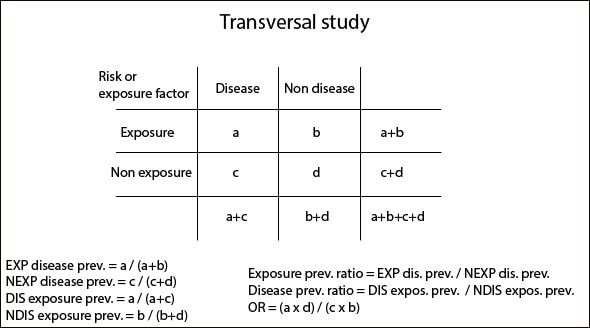The first we’re going to talk about are cross-sectional studies’ tables. This type of study represents a sort of snapshot of our sample that allows us to study the relationship between the variables. They’re, therefore, prevalence studies and, although data can be collected over a period of time, the result only represents the snapshot we have already mentioned. Dependent variable is placed in columns (disease status) and independent variable in rows (exposure status), so we can calculate a series of frequency, association and statistical significance measures.

The frequency measures are the prevalence of disease among exposed (EXP) and unexposed (NEXP) and the prevalence of exposure among diseased (DIS) and non-diseased (NDIS). These prevalences represent the number of sick, healthy, exposed and unexposed in relation to each group total, so they are rates estimated in a precise moment.

The measures of association are the rates between prevalences just aforementioned according to exposure and disease status, and the odds ratio, which tells us how much more likely the disease will occur in exposed (EXP) versus non-exposed (NEXP) people. If these parameters have a value greater than one it will indicate that the exposure factor is a risk factor for disease. On the contrary, a value equal or greater than zero and less than one will mean a protective factor. And if the value equals one, it will be neither fish nor fowl.

Finally, as in all types of tables that we’ll mention, you can calculate statistical significance measures, mainly chi-square with or without correction, Fisher’s exact test and p value, unilateral or bilateral.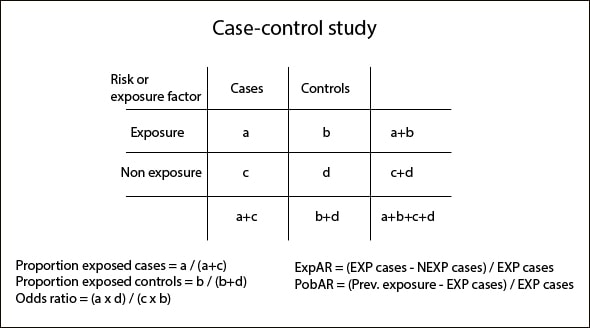Very much like those table we’ve just seen are case-control studies’ tables. This study design tries to find out if different levels of exposure can explain different levels of disease. Cases and controls are placed in columns and exposure status (EXP and NEXP) in rows.

The measures of frequency that we can calculate are the proportion of exposed cases (based on the total number of cases) and the proportion of exposed controls (based on the total number of controls). Obviously, we can also come up with the proportions of non-exposed calculating the complementary values of the aforementioned ones.

The key measure of association is the odds ratio that we already know and in which we are not going to spend much time. All of us know that, in the simplest way, we can calculate its value as the ratio of the cross products of the table and that it informs us about how much more likely is the disease to occur in exposed than in non-exposed people. The other measure of association is the exposed attributable fraction (ExpAR), which indicates the number of patients who are sick due to direct effect of exposition.

Managing this type of tables, we can also calculate a measure of impact: the population attributable fraction (PopAR), which tells us what would happen on the population if we eliminated the exposure factor. If the exposure factor is a risk factor, the impact will be positive. Conversely, if we are dealing with a protective factor, its elimination impact will be negative.

With this type of study design, the statistical significance measures will be different if we are managing paired (McNemar test) or un-paired data (chi-square, Fisher’s exact test and p value).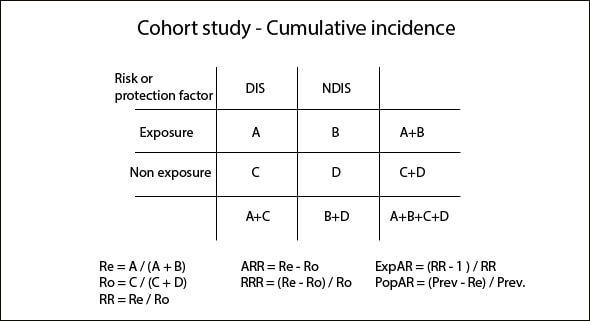The third type of contingency tables is the corresponding to cohort studies, although their structure differ slightly if you count total cases along the entire period of the study (cumulative incidence) or if you consider the time period of the study, the time of onset of disease in cases and the different time of follow-up among groups (incidence rate or incidence density).

Tables from cumulative incidence studies (CI) are similar to those we have seen so far. Disease status is represented in columns and exposure status in rows. Otherwise, incidence density (ID) tables represent in the first column the number of patients and, in the second column, the follow-up in patients-years format, so that those with longer follow-up have greater weight when calculating measures of frequency, association, etc.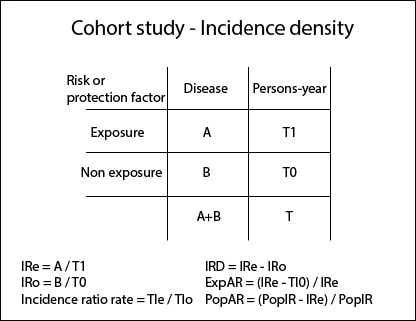The measures of frequency are the EXP risk (Re) and the NEXP risk (Ro) for CI studies and EXP and NEXP incidence rates in ID studies.

We can calculate the ratios of the above measures to come up with the association measures: relative risk (RR), absolute risk reduction (ARR) and relative risk reduction (RRR) for CI studies and incidence density reduction (IRD) for ID studies. In addition, we can also calculate ExpAR as we did in the cases-control study, as well as a measure of impact: PopAR.

We can also calculate the odds ratios if we want, but they are generally much less used in this type of study design. In any case, we know that RR and odds ratio are very similar when disease prevalence is low.

To end with this kind of table, we can calculate the statistical significance measures: chi-square, Fisher’s test and p value for CI studies and other association measures for ID studies.

As always, all these calculations can be done by hand, although I recommend you to use a calculator, such as the available one at the CASPe site. It’s easier and faster and further we will come up with all these parameters and their confidence intervals, so we can also estimate their precision.

And with this we come to the end. There’re more types of tables, with multiple levels for managing more than two variables, stratified according to different factors and so on. But that’s another story…